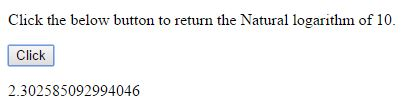# Natural Logarithm (LN) in JavaScript

#### LN Property (Natural Logarithm)

The natural logarithm of a number is its logarithm to the base e, where e is irrational and transcendental constant. The natural logarithm of x is generally written as loge x, ln x OR loge(x), ln(x).

In JavaScript, by using `LN` property we can return the Natural logarithm value.

### Returning the value of `LN2`(Natural Logarithm of 2).

```<p>Click the below button to return the Natural logaritham of 2.</p>
<input type="button" onclick="myNumber()" value="Click">
<p id="myId"></p>

<script>
function myNumber() {
document.getElementById("myId").innerHTML = Math.LN2;
}
</script>
```

In the above code snippet we have given `Id` as "`myId`"to the second `<p>` element in the HTML code. There is a function myNumber() in the`<script>`block which is connected to the onclick of the HTML button. We need to return the natural logarithm of 2, for that we are using `LN2`property. Onclick of the button "Click" in the HTML code fires the function myNumber() in the `<script>` block, at the same time `Math.LN2` property return the natural logarithm of 2 value as output.

OUTPUT### Returning the value of `LN10`.

```<p>Click the below button to return the Natural logaritham of 10.</p>
<input type="button" onclick="myNumber()" value="Click">
<p id="myId"></p>

<script>
function myNumber() {
document.getElementById("myId").innerHTML = Math.LN10;
}
</script>
```

Logarithm of 10

Onclick of the button "Click" in the HTML code fires the function myNumber() in the `<script>` block, at the same time `Math.LN10` property return the natural logarithm of 10 value as output.

OUTPUTNOTE: The `LN` property consits of only 2 parameters, i.e `LN2 `& `LN10`.

Views: 7504 | Post Order: 92